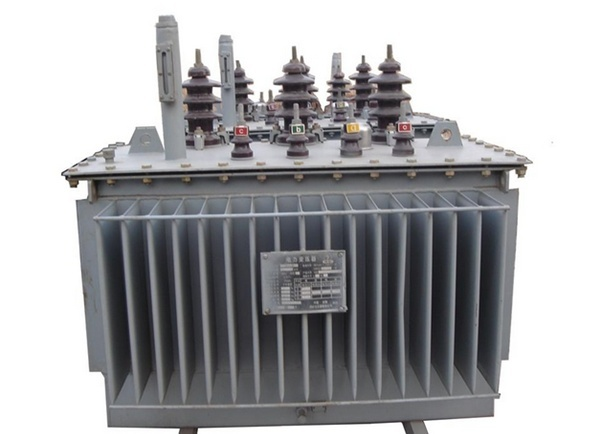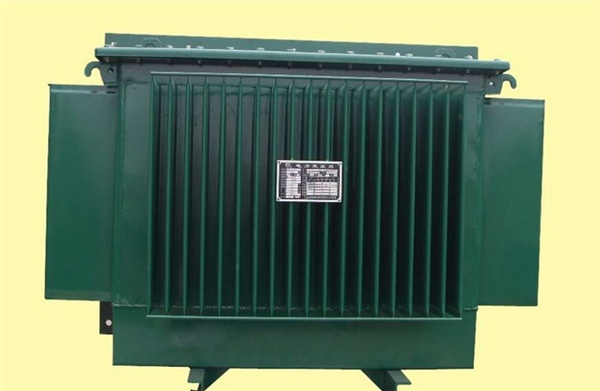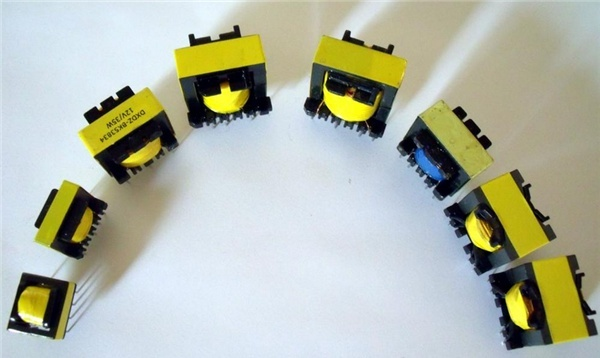|

# 变压器原理是什么 变压器分类

在用电，多数用电器所需电压是380V、220V或36V，少数电机也采用3kV、6kV等。电能输送到用电后，适应用电设备的电压，还需通过各级变电站（所）变压器将电压降低为各类电器所的电压值。这时就需要变压器来转换电伏了，那变压器原理是什么呢？什么是是变压器

变压器是变换交流电压、电流和阻抗的器件，当初级线圈中通有交流电流时，铁芯（或磁芯）中便产生交流磁通，使次级线圈中感应出电压（或电流）。变压器由铁芯（或磁芯）和线圈组成，线圈有两个或两个的绕组，其中接电源的绕组叫初级线圈，其余的绕组叫次级线圈。

变压器是一种的电子元件和电气设备，来把某种数值的交变电压变换为同频率的另一数值的交变电压，也改变交流电的数值及变换阻抗或改变相位或变换频率。发电厂欲将P＝sqrt（3）＊UIcosφ的电功率输送到用电的，在P、cosφ为值时，若采用的电压愈高，则输电线路中的电流愈小，因而减少输电线路上的损耗，节约导电材料。远距离输电采用高电压是最为经济的。目前，我国交流输电的电压最高已达500kV。高的电压，无论从发电机的安全运行或是从制造成本考虑，都不允许由发电机直接生产。发电机的输出电压有3.15kV、6.3kV、10.5kV、15.75kV等几种，用升压变压器将电压升高才能远距离输送。变压器的工作原理

变压器由铁芯（或磁芯）和线圈组成，线圈有两个或两个以上的绕组，其中接电源的绕组叫初级线圈，其余的绕组叫次级线圈。它可以变换交流电压、电流和阻抗。最简单的铁心变压器由一个软磁材料做成的铁心及套在铁心上的两个匝数不等的线圈构成。

铁心的作用是加强两个线圈间的磁耦合。为了减少铁内涡流和磁滞损耗，铁心由涂漆的硅钢片叠压而成；两个线圈之间没有电的联系，线圈由绝缘铜线（或铝线）绕成。一个线圈接交流电源称为初级线圈（或原线圈），另一个线圈接用电器称为次级线圈（或副线圈）。实际的变压器是很复杂的，不可避免地存在铜损（线圈电阻发热）、铁损（铁心发热）和漏磁（经空气闭合的磁感应线）等，为了简化讨论这里只介绍理想变压器。理想变压器成立的条件是：忽略漏磁通，忽略原、副线圈的电阻，忽略铁心的损耗，忽略空载电流（副线圈开路原线圈线圈中的电流）。例如电力变压器在满载运行时（副线圈输出额定功率）即接近理想变压器情况。变压器分类

1、起电压和功率变换作用的电源变压器，功率变压器，整流变压器，逆变变压器，开关变压器，脉冲功率变压器；

2、起传递宽带、声频、中周功率和信号作用的宽带变压器，声频变压器，中周变压器；

3、起传递脉冲、驱动和触发信号作用的脉冲变压器，驱动变压器，触发变压器；

4、起原边和副边绝缘隔离作用的隔离变压器，起屏蔽作用的屏蔽变压器；

5、起单相变三相或三相变单相作用的相数变换变压器，起改变输出相位作用的相位变换变压器（移相器）；

6、起改变输出频率作用的倍频或分频变压器；

7、起改变输出阻抗与负载阻抗相匹配作用的匹配变压器；

8、起稳定输出电压或电流作用的稳压变压器（包括恒压变压器）或稳流变压器，起调节输出电压作用的调压变压器；

9、起交流和直流滤波作用的滤波电感器；

10、起抑制电磁干扰作用的电磁干扰滤波电感器，起抑制噪声作用的噪声滤波电感器；

11、起吸收浪涌电流作用的吸收电感器，起减缓电流变化速率的缓冲电感器；

12、起储能作用的储能电感器，起帮助半导体开关换向作用的换向电感器；

13、起开关作用的磁性开关电感器和变压器；

14、起调节电感作用的可控电感器和饱和电感器；

15、起变换电压、电流或脉冲检测信号的电压互感器、电流互感器、脉冲互感器、直流互感器、零磁通互感器、弱电互感器、零序电流互感器、霍尔电流电压检测器。不论是直流电源，交流电源，还是特种电源，都离不开电子变压器。有人把电源界定为经过高频开关变换的直流电源和交流电源。在介绍软磁电磁元件在电源技术中的作用时，往往举高频开关电源中的各种电磁元件为例证。

`声明：本文由入驻焦点开放平台的作者撰写，除焦点官方账号外，观点仅代表作者本人，不代表焦点立场错误信息举报电话： 400-099-0099，邮箱：jubao@vip.sohu.com，或点此进行意见反馈，或点此进行举报投诉。`A B C D E F G H J K L M N P Q R S T W X Y Z
A - B - C - D - E
• A
• 鞍山
• 安庆
• 安阳
• 安顺
• 安康
• 澳门
• B
• 北京
• 保定
• 包头
• 巴彦淖尔
• 本溪
• 蚌埠
• 亳州
• 滨州
• 北海
• 百色
• 巴中
• 毕节
• 保山
• 宝鸡
• 白银
• 巴州
• C
• 承德
• 沧州
• 长治
• 赤峰
• 朝阳
• 长春
• 常州
• 滁州
• 池州
• 长沙
• 常德
• 郴州
• 潮州
• 崇左
• 重庆
• 成都
• 楚雄
• 昌都
• 慈溪
• 常熟
• D
• 大同
• 大连
• 丹东
• 大庆
• 东营
• 德州
• 东莞
• 德阳
• 达州
• 大理
• 德宏
• 定西
• 儋州
• 东平
• E
• 鄂尔多斯
• 鄂州
• 恩施
F - G - H - I - J
• F
• 抚顺
• 阜新
• 阜阳
• 福州
• 抚州
• 佛山
• 防城港
• G
• 赣州
• 广州
• 桂林
• 贵港
• 广元
• 广安
• 贵阳
• 固原
• H
• 邯郸
• 衡水
• 呼和浩特
• 呼伦贝尔
• 葫芦岛
• 哈尔滨
• 黑河
• 淮安
• 杭州
• 湖州
• 合肥
• 淮南
• 淮北
• 黄山
• 菏泽
• 鹤壁
• 黄石
• 黄冈
• 衡阳
• 怀化
• 惠州
• 河源
• 贺州
• 河池
• 海口
• 红河
• 汉中
• 海东
• I
• J
• 晋中
• 锦州
• 吉林
• 鸡西
• 佳木斯
• 嘉兴
• 金华
• 景德镇
• 九江
• 吉安
• 济南
• 济宁
• 焦作
• 荆门
• 荆州
• 江门
• 揭阳
• 金昌
• 酒泉
• 嘉峪关
K - L - M - N - P
• K
• 开封
• 昆明
• 昆山
• L
• 廊坊
• 临汾
• 辽阳
• 连云港
• 丽水
• 六安
• 龙岩
• 莱芜
• 临沂
• 聊城
• 洛阳
• 漯河
• 娄底
• 柳州
• 来宾
• 泸州
• 乐山
• 六盘水
• 丽江
• 临沧
• 拉萨
• 林芝
• 兰州
• 陇南
• M
• 牡丹江
• 马鞍山
• 茂名
• 梅州
• 绵阳
• 眉山
• N
• 南京
• 南通
• 宁波
• 南平
• 宁德
• 南昌
• 南阳
• 南宁
• 内江
• 南充
• P
• 盘锦
• 莆田
• 平顶山
• 濮阳
• 攀枝花
• 普洱
• 平凉
Q - R - S - T - W
• Q
• 秦皇岛
• 齐齐哈尔
• 衢州
• 泉州
• 青岛
• 清远
• 钦州
• 黔南
• 曲靖
• 庆阳
• R
• 日照
• 日喀则
• S
• 石家庄
• 沈阳
• 双鸭山
• 绥化
• 上海
• 苏州
• 宿迁
• 绍兴
• 宿州
• 三明
• 上饶
• 三门峡
• 商丘
• 十堰
• 随州
• 邵阳
• 韶关
• 深圳
• 汕头
• 汕尾
• 三亚
• 三沙
• 遂宁
• 山南
• 商洛
• 石嘴山
• T
• 天津
• 唐山
• 太原
• 通辽
• 铁岭
• 泰州
• 台州
• 铜陵
• 泰安
• 铜仁
• 铜川
• 天水
• 天门
• W
• 乌海
• 乌兰察布
• 无锡
• 温州
• 芜湖
• 潍坊
• 威海
• 武汉
• 梧州
• 渭南
• 武威
• 吴忠
• 乌鲁木齐
X - Y - Z
• X
• 邢台
• 徐州
• 宣城
• 厦门
• 新乡
• 许昌
• 信阳
• 襄阳
• 孝感
• 咸宁
• 湘潭
• 湘西
• 西双版纳
• 西安
• 咸阳
• 西宁
• 仙桃
• 西昌
• Y
• 运城
• 营口
• 盐城
• 扬州
• 鹰潭
• 宜春
• 烟台
• 宜昌
• 岳阳
• 益阳
• 永州
• 阳江
• 云浮
• 玉林
• 宜宾
• 雅安
• 玉溪
• 延安
• 榆林
• 银川
• Z
• 张家口
• 镇江
• 舟山
• 漳州
• 淄博
• 枣庄
• 郑州
• 周口
• 驻马店
• 株洲
• 张家界
• 珠海
• 湛江
• 肇庆
• 中山
• 自贡
• 资阳
• 遵义
• 昭通
• 张掖
• 中卫

1室1厅1厨1卫1阳台

1
2
3
4
5

0
1
2

1

1

0
1
2
3报名成功，资料已提交审核A B C D E F G H J K L M N P Q R S T W X Y Z
A - B - C - D - E
• A
• 鞍山
• 安庆
• 安阳
• 安顺
• 安康
• 澳门
• B
• 北京
• 保定
• 包头
• 巴彦淖尔
• 本溪
• 蚌埠
• 亳州
• 滨州
• 北海
• 百色
• 巴中
• 毕节
• 保山
• 宝鸡
• 白银
• 巴州
• C
• 承德
• 沧州
• 长治
• 赤峰
• 朝阳
• 长春
• 常州
• 滁州
• 池州
• 长沙
• 常德
• 郴州
• 潮州
• 崇左
• 重庆
• 成都
• 楚雄
• 昌都
• 慈溪
• 常熟
• D
• 大同
• 大连
• 丹东
• 大庆
• 东营
• 德州
• 东莞
• 德阳
• 达州
• 大理
• 德宏
• 定西
• 儋州
• 东平
• E
• 鄂尔多斯
• 鄂州
• 恩施
F - G - H - I - J
• F
• 抚顺
• 阜新
• 阜阳
• 福州
• 抚州
• 佛山
• 防城港
• G
• 赣州
• 广州
• 桂林
• 贵港
• 广元
• 广安
• 贵阳
• 固原
• H
• 邯郸
• 衡水
• 呼和浩特
• 呼伦贝尔
• 葫芦岛
• 哈尔滨
• 黑河
• 淮安
• 杭州
• 湖州
• 合肥
• 淮南
• 淮北
• 黄山
• 菏泽
• 鹤壁
• 黄石
• 黄冈
• 衡阳
• 怀化
• 惠州
• 河源
• 贺州
• 河池
• 海口
• 红河
• 汉中
• 海东
• I
• J
• 晋中
• 锦州
• 吉林
• 鸡西
• 佳木斯
• 嘉兴
• 金华
• 景德镇
• 九江
• 吉安
• 济南
• 济宁
• 焦作
• 荆门
• 荆州
• 江门
• 揭阳
• 金昌
• 酒泉
• 嘉峪关
K - L - M - N - P
• K
• 开封
• 昆明
• 昆山
• L
• 廊坊
• 临汾
• 辽阳
• 连云港
• 丽水
• 六安
• 龙岩
• 莱芜
• 临沂
• 聊城
• 洛阳
• 漯河
• 娄底
• 柳州
• 来宾
• 泸州
• 乐山
• 六盘水
• 丽江
• 临沧
• 拉萨
• 林芝
• 兰州
• 陇南
• M
• 牡丹江
• 马鞍山
• 茂名
• 梅州
• 绵阳
• 眉山
• N
• 南京
• 南通
• 宁波
• 南平
• 宁德
• 南昌
• 南阳
• 南宁
• 内江
• 南充
• P
• 盘锦
• 莆田
• 平顶山
• 濮阳
• 攀枝花
• 普洱
• 平凉
Q - R - S - T - W
• Q
• 秦皇岛
• 齐齐哈尔
• 衢州
• 泉州
• 青岛
• 清远
• 钦州
• 黔南
• 曲靖
• 庆阳
• R
• 日照
• 日喀则
• S
• 石家庄
• 沈阳
• 双鸭山
• 绥化
• 上海
• 苏州
• 宿迁
• 绍兴
• 宿州
• 三明
• 上饶
• 三门峡
• 商丘
• 十堰
• 随州
• 邵阳
• 韶关
• 深圳
• 汕头
• 汕尾
• 三亚
• 三沙
• 遂宁
• 山南
• 商洛
• 石嘴山
• T
• 天津
• 唐山
• 太原
• 通辽
• 铁岭
• 泰州
• 台州
• 铜陵
• 泰安
• 铜仁
• 铜川
• 天水
• 天门
• W
• 乌海
• 乌兰察布
• 无锡
• 温州
• 芜湖
• 潍坊
• 威海
• 武汉
• 梧州
• 渭南
• 武威
• 吴忠
• 乌鲁木齐
X - Y - Z
• X
• 邢台
• 徐州
• 宣城
• 厦门
• 新乡
• 许昌
• 信阳
• 襄阳
• 孝感
• 咸宁
• 湘潭
• 湘西
• 西双版纳
• 西安
• 咸阳
• 西宁
• 仙桃
• 西昌
• Y
• 运城
• 营口
• 盐城
• 扬州
• 鹰潭
• 宜春
• 烟台
• 宜昌
• 岳阳
• 益阳
• 永州
• 阳江
• 云浮
• 玉林
• 宜宾
• 雅安
• 玉溪
• 延安
• 榆林
• 银川
• Z
• 张家口
• 镇江
• 舟山
• 漳州
• 淄博
• 枣庄
• 郑州
• 周口
• 驻马店
• 株洲
• 张家界
• 珠海
• 湛江
• 肇庆
• 中山
• 自贡
• 资阳
• 遵义
• 昭通
• 张掖
• 中卫• 手机• 分享
• 设计
免费设计
• 计算器
装修计算器
• 入驻
合作入驻
• 联系
联系我们
• 置顶
返回顶部